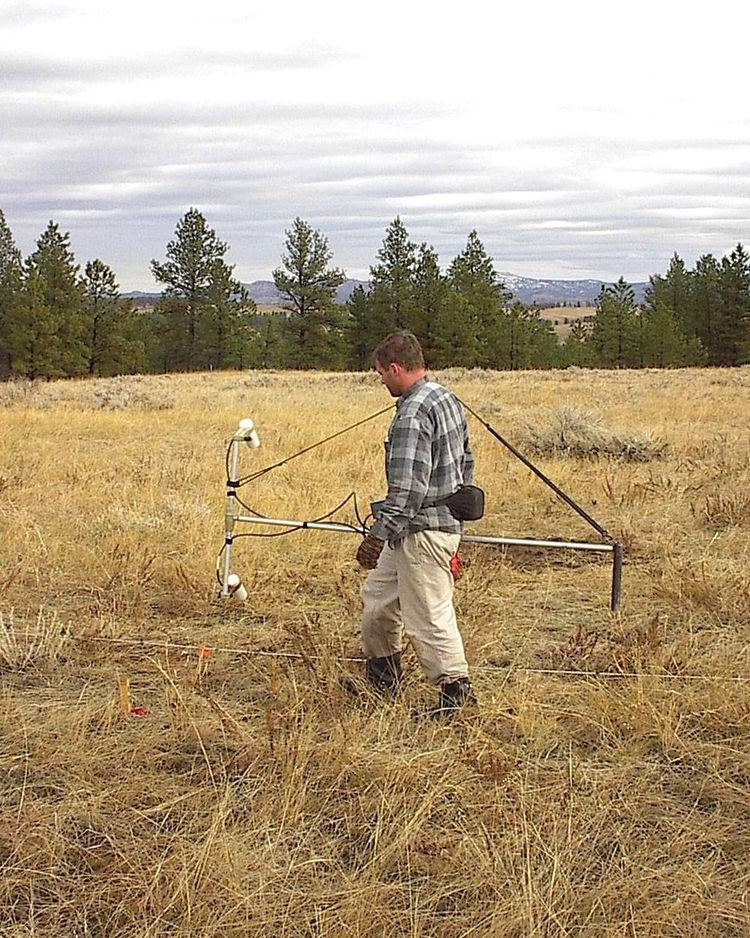Updated onA gradiometer measures the gradient (numerical rate of change) of a physical quantity, such as a magnetic field or gravity.

There are at least two types of gradiometer to measure magnetic fields:

1. Axial gradiometer. Such a device consists of two magnetometers placed in series (i.e. one above the other). The result coming from the device is the difference in magnetic flux at that point in space, or in other words, the result is the difference between what each of the magnetometers see.
2. Planar gradiometer. Such a device consists of two magnetometers placed next to each other. The result coming from the device is the difference in flux between the two loops.

Each sensor type responds differently to certain spatial signals.

Axial gradiometers are good for measuring depth and planar ones can measure the signal even from a small exposure, ie they can measure signals even under a lot of noise.

Topics